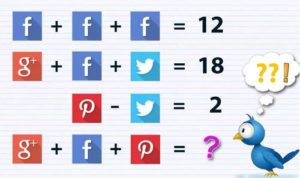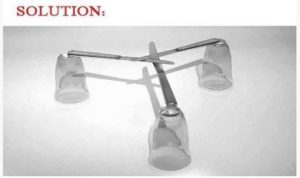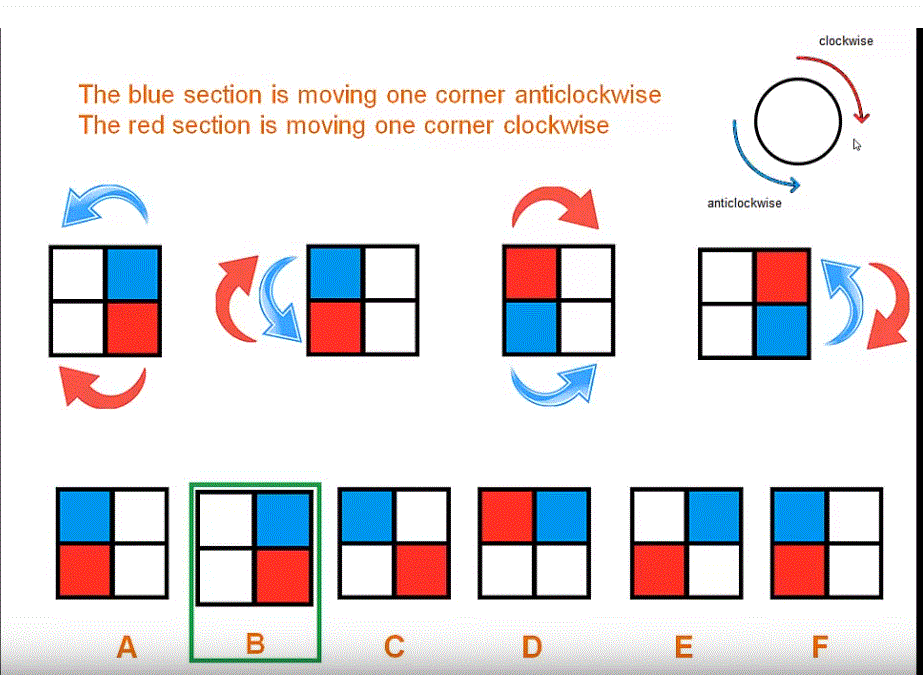Select Page

November 2018

## Did you try each several times?

Here are the answers to Brain Practice, November 2018

#### Social Media ChallengeHow did we reach that?

Now our puzzle question becomes:
⇒ F + F + F = 12
⇒ G + F + T = 18
⇒ P – T = 2
⇒ G + F + P = ??

### ♣ 1st Equation:

3F = 12   ⇒   F = 12/3   ⇒    F = 4

### ♣ 2nd Equation:

G + F + T = 18    ⇒    G + 4 + T = 18    ⇒     G + T = 18 – 4    ⇒    G + T = 14
(Now, as you can see we can’t solve this further yet, but let’s try next equation)

### ♣ 3rd Equation:

P – T = 2
(Now compare/add this equation with the 2nd equation i.e G + T = 14)
G + T + P – T = 14 + 2    (+T and -T cancel out each other and we get)
G + P = 16

### ♣ 4th Equation:

G + F + P = ??
We don’t know the individual values of G & P here, but we know G + P = 16 (from 3rd equation) & F = 4 (from 1st equation), put their value in the 4th equation and you will get the answer.
(G + P) + F = ??    ⇒   16 + 4  ⇒  20 Answer

#### How Old is Granny?

Tom’s Granny is 58 years old.

Let’s see why:

First child born: Granny is 19
Second child born: Granny is 23 (19 + 4)
Third child born: Granny is 27 (23 + 4)
Fourth child born: Granny is 31 (27 + 4)
Fifth child born: Granny is 35 (31 + 4)
Sixth child born: Granny is 39 (35 + 4)
Sixth child is 19: Granny is 58 (39 + 19)

#### Build a bridge with three glasses and three knives?#### Solve these Riddles?

What building has the most stories? A library of course!
If two’s company and three’s a crowd, what’s four and five? Nine

If you throw a white stone into the Red Sea, what would it become? Wet

(I know you are groaning! )

#### Complete the Sequence